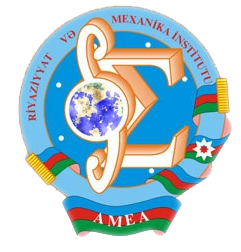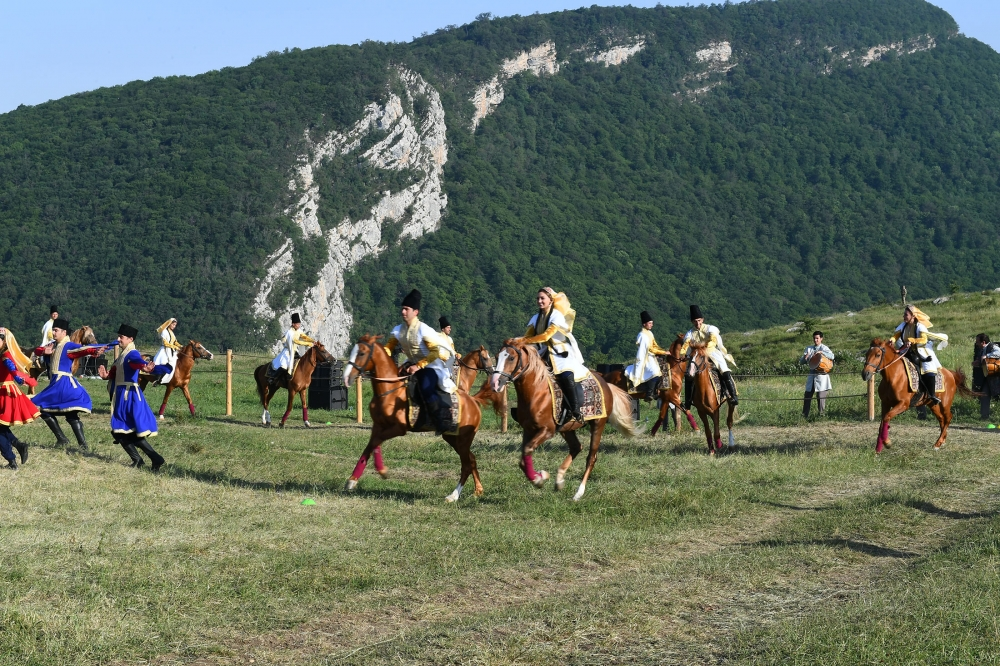Riyaziyyat və Mexanika İnstitutu

## Qarabağ xəbərləri### Şuşada Heydər Əliyev Fondunun təşkilatçılığı ilə “Musiqi irsi və Qarabağ atları Cıdır düzündə” adlı kompozisiya təqdim olunub## Proceedings 2004/21/XXIX

 Year: 2004 Volume: XXI Number: XXIX MATHEMATICS Akhundov A.Ya. Abstract [PDF] The inverse problems for one system of parabolic equations [PDF] 3 Aliyev R.M, Gasymova S.G. Abstract [PDF] On quadrature formulae [PDF] 17 Aslanov Kh.M. Abstract [PDF] Stability of the inverse problem of scattering theory of Sturm-Liouville self-adjoint operator [PDF] 27 Babayev A.A. Abstract [PDF] Simplification of an algorithm for recognition of equality of canonical morphisms in the categories with additional structures [PDF] 39 Babayev Sh.A. Abstract [PDF] Investigation of the boundary functional of the semi-Markov process with negative drift and delaying screen in the zero [PDF] 45 Bilalov B.T. Abstract [PDF] On a Stone – Weierstrass theorem [PDF] 51 Gasimova E.R. Abstract [PDF] Strong solvability of the first boundary value problem for degenerate elliptic-parabolic equations of second order [PDF] 55 Guseynov F.B. Abstract [PDF] A mixed problem for Barenblatt-Jeltov-Kochina equation in bounded domain [PDF] 71 Hajibayov M.G. Abstract [PDF] On the weak differentiability of anisotropic Riesz potentials [PDF] 85 Huseynbayova A.M. Abstract [PDF] Investigation of the existence conditions and finding the number of stationary states of quantum mechanical particles in the narrow deep potential pit [PDF] 95 Imranov F.B. Abstract [PDF] On the existence of solution of difference scheme for a stationary problem of incompressible liquid with non-zero boundary conditions [PDF] 105 Khalilov R.F. Abstract [PDF] On the best approximation of complex variable function in infinite domains [PDF] 117 Kuliyev H.F., Teyyubiva F.R. Abstract [PDF] The optimal control problem for the equation of oscillations of a three-layer plate [PDF] 121 Maharov I.K. Abstract [PDF] An approximate characterization of vector-valued Besov spaces defined by differences of fraction order [PDF] 131 Mayorova I.V. Abstract [PDF] To estimates of Lq -traces of functions and their derivatives on boundary m- dimensional surface [PDF] 141 Mirzoyev S.S., Veliyev S.G. Abstract [PDF] On basicity of the system of exponents in the weight space [PDF] 149 Namazov G.K., Mehraliyev Y.T. Abstract [PDF] The inverse boundary value problem for a higher order nonlinear pseudoparabolic equation with nonself-adjoint boundary conditions [PDF] 151 Piriyeva A.E. Abstract [PDF] On order of approximation of functions by generalized Bernstein- Chlodowsky polynomials [PDF] 157 Rzayev K.U. Abstract [PDF] An application of Tikhonov’s method to the proof of the existence of the solution of uncountable system of partial differential equations [PDF] 165 Sabzaliyev M.M. Abstract [PDF] The asymptotic form of solution of the boundary value problem for singular perturbed quasilinear parabolic differential equation [PDF] 169 Sadygov M.A. Abstract [PDF] On the characterization of zeros and fixed point of mappings [PDF] 177 Yusufov V.Sh. Abstract [PDF] Open and open-covering mappings of topological spaces [PDF] 187 MECHANICS Aliyev I.Ya. Abstract [PDF] Boundary crack in I type two-play orthotropic materials [PDF] 193 Aqalarova N.J. Abstract [PDF] The solution of a task on propagation of waves on a surface of a liquid due to movement of the cylinder [PDF] 199 Babanli V.Yu. Abstract [PDF] Nonstationary filtrarion of two-phase system involving impermeable barrier [PDF] 203 Giyasbeyli S.A. Abstract [PDF] The asymptotic analysis of eigenfrequencies of oscillations of the cylindrical envelopes, strengthened by transverse ribs and filled with fluid [PDF] 209 Hassanov H.G. Abstract [PDF] Hydraulic impact of compressible viscous liquid [PDF] 213 Mir-Salim-Zadeh M.V. Abstract [PDF] Crack with bondings between faces in rib reinforced [PDF] 219 APPLIED PROBLEMS OF MATHEMATICS AND MECHANICS Melikov T.K. Abstract [PDF] On conditions of Kelley type optimality in Goursat-Dauboux systems [PDF] 229 Muslumov V.B. Abstract [PDF] Second order necessary optimality conditions in one boundary control problem [PDF] 237 Nadjafov T.I. Abstract [PDF] A problem on periodic trajectories of circular dynamic billiard and its applications [PDF] 245 Panahov G.M., Agayeva G.R. Abstract [PDF] Control of nonstationary processes at formation of complex hardening systems [PDF] 253
Azərbaycanda COVID-19 ilə bağlı statistika
• Virusa yoluxan

596973

• Sağalan

565521

• Yeni yoluxan

0

• Aktiv xəstə

23469

• Ölüm halı

7983

• Test edilib

5,608,158